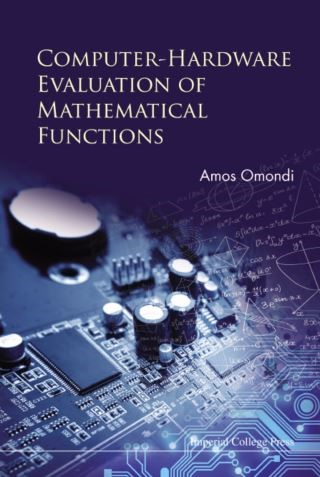## Computer-hardware Evaluation Of Mathematical Functions• Description
• Author
• Info
• Reviews

### Description

Computer-Hardware Evaluation of Mathematical Functions provides a thorough up-to-date understanding of the methods used in computer hardware for the evaluation of mathematical functions: reciprocals, square-roots, exponentials, logarithms, trigonometric functions, hyperbolic functions, etc. It discusses how the methods are derived, how they work, and how well they work. The methods are divided into four core themes: CORDIC, normalization, table look-up, and polynomial approximations. In each case, the author carefully considers the mathematical derivation and basis of the relevant methods, how effective they are (including mathematical errors analysis), and how they can be implemented in hardware.

This book is an excellent resource for any student or researcher seeking a comprehensive, yet easily understandable, explanation of how computer chips evaluate mathematical functions.

Computer-Hardware Evaluation of Mathematical Functions provides a thorough up-to-date understanding of the methods used in computer hardware for the evaluation of mathematical functions: reciprocals, square-roots, exponentials, logarithms, trigonometric functions, hyperbolic functions, etc. It discusses how the methods are derived, how they work, and how well they work. The methods are divided into four core themes: CORDIC, normalization, table look-up, and polynomial approximations. In each case, the author carefully considers the mathematical derivation and basis of the relevant methods, how effective they are (including mathematical errors analysis), and how they can be implemented in hardware.

This book is an excellent resource for any student or researcher seeking a comprehensive, yet easily understandable, explanation of how computer chips evaluate mathematical functions.

Readership: Graduate and undergraduate students and researchers interested in the hardware and software aspects of computer chips.
Key Features:
• First full-length book on the subject
• Contains up-to-date information
• Detailed and easy to use